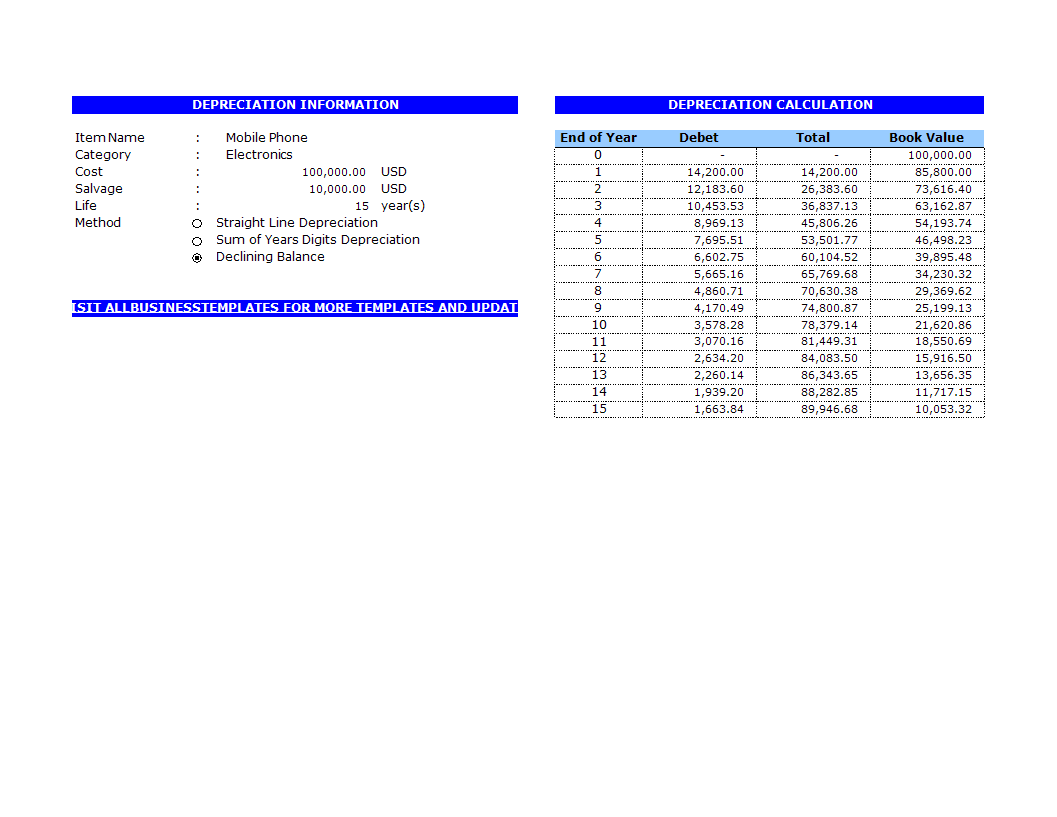# Depreciation Calculator

Save, fill-In The Blanks, Print, Done!Click on image to zoom / Click button below to see more images

Or select the format you want and we convert it for you for free:

• This Document Has Been Certified by a Professional
• 100% customizable
• This is a digital download (41.5 kB)
• Language: English

ABT template rating: 7

Malware- and virusfree. Scanned by:How to make a Depreciation Calculator  in Excel? An easy way to create your spreadsheet is by downloading this example Depreciation Calculator Excel spreadsheet template now!

The depreciation calculator is for depreciation calculation in accounting. It takes straight line, declining balance, or sum of the year' digits method. If you are using double declining balance method, just select declining balance and set the depreciation factor to be 2. It can also calculate partial-year depreciation with any accounting year date setting.

Depreciation
Conceptually, depreciation is the reduction in value of an asset over time, due to elements such as wear and tear. For instance, a widget-making machine is said to "depreciate" when it produces less widgets one year compared to the year before it, or a car is said to "depreciate" in value after a fender bender or the discovery of a faulty transmission.

For accounting in particular, depreciation concerns allocating the cost of an asset over a period of time, usually its useful life. When a company purchases an asset, such as a piece of equipment, such large purchases can skewer the income statement confusingly. Instead of appearing as a sharp jump in the accounting books, this can be smoothed by expensing the asset over its useful life. Within a business in the U.S., depreciation expenses are tax-deductible.

Methods of Depreciation
There are many methods of distribution depreciation amount over its useful life. The following are some of the widely used methods. The total amount of depreciation for any asset will be identical in the end no matter which method of depreciation is chosen; only the timing of depreciation will be altered. Keep in mind that accelerated depreciation methods (such as declining balance or sum of the years' digits) can artificially reduce profit in the near term followed by higher profits in later terms, which can influence reported cash flows.

Straight-Line Depreciation Method
Straight-line depreciation is the most widely used and simplest method. It is a method of distributing the cost evenly across the useful life of the asset. The following is the formula:

Depreciation per year =
Asset Cost - Salvage Value
Useful life
Declining Balance Depreciation Method
For specific assets, the newer they are, the faster they depreciate in value. As these assets age, their depreciation rates slow over time. In these situations, the declining balance method tends to be more accurate than the straight-line method at reflecting book value each year.

Depreciation per year = Book value × Depreciation rate

Double declining balance is the most widely used declining balance depreciation method, which has a depreciation rate that is twice the value of straight line depreciation for the first year. Use a depreciation factor of two when doing calculations for double declining balance depreciation. Regarding this method, salvage values are not included in the calculation for annual depreciation. However, depreciation stops once book values drop to salvage values.

Sum of the Years' Digits Depreciation Method
Similar to declining balance depreciation, sum of the years' digits (SYD) depreciation also results in faster depreciation when the asset is new. It is generally more useful than straight-line depreciation for certain assets that have greater ability to produce in the earlier years, but tend to slow down as they age.

Depreciation for the Year = (Asset Cost - Salvage Value) × factor

1st year: factor = n / (1+2+3+...+ n)
2nd year: factor = (n-1) / (1+2+3+...+ n)
3rd year: factor = (n-2) / (1+2+3+...+ n)
...
last year: factor = 1 / (1+2+3+...+ n)

n is the asset's useful life in years.

Our Excel templates are grid-based files designed to organize information and perform calculations with scalable entries. Beginners and professionals from all over the world are now using spreadsheets to create tables, calculations, comparisons, overviews, etc for any personal or business need.

This Excel template is a great way to increase your productivity and performance. It gives you access to do remarkable new things with Excel, even if you only have a basic understanding of working with formula’s and spreadsheets. If time or quality is of the essence, this ready-made presentation can certainly help you out!

DISCLAIMER
Nothing on this site shall be considered legal advice and no attorney-client relationship is established.

Leave a Reply. If you have any questions or remarks, feel free to post them below.## How do I make an Infographic?

### Latest topics

More topics

One finds limits by pushing them. | Herbert Simon